7 篇文章 4 订阅

哈夫曼树

设二叉树具有n个带权值的叶节点，那么从根节点到各个叶节点的路径长度与相应节点权值的乘积的和，叫做二叉树的带权路径长度。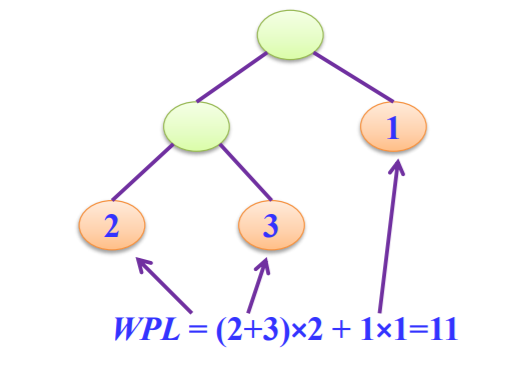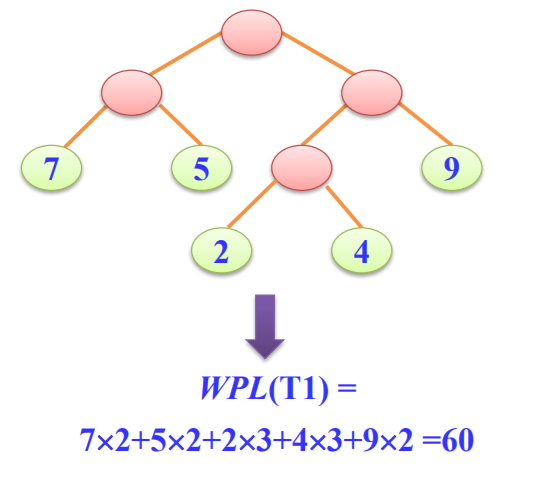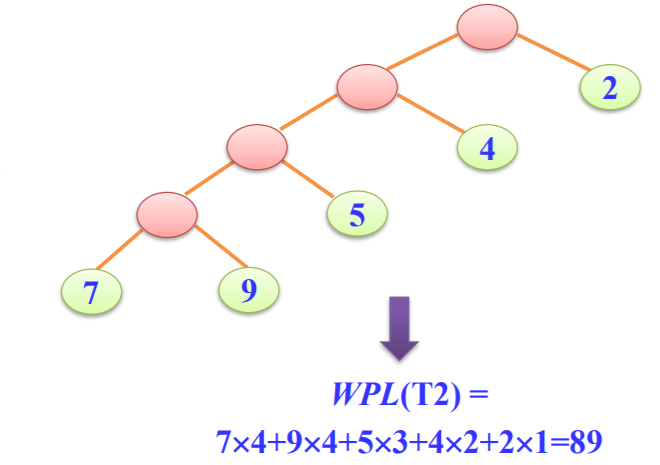哈夫曼树的构造：

原则：

• 权值越大的叶节点越靠近根节点。
• 权值越小的叶节点越远离根节点。

过程：

（1）给定的n个权值{W1,W2,...,Wn }构造n棵只有一个叶节点的二叉树， 从而得到一个二叉树的集合F={T1，T2，…，Tn }。

（2）在F中选取根节点的权值最小和次小的两棵二叉树作为左、右子树 构造一棵新的二叉树，这棵新的二叉树根节点的权值为其左、右子树根节点 权值之和。

（3）在集合F中删除作为左、右子树的两棵二叉树，并将新建立的二叉 树加入到集合F中。

（4）重复（2）、（3）两步，当F中只剩下一棵二叉树时，这棵二叉树 便是所要建立的哈夫曼树。

示例：（5，29，7，8，14，23，3，11）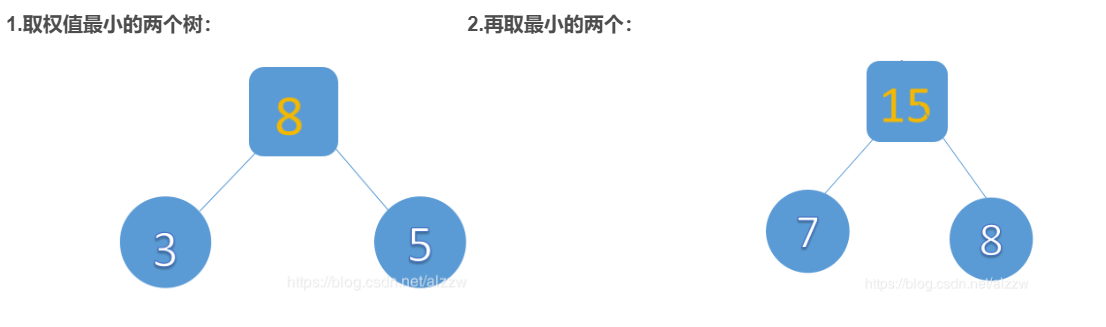3.以此类推：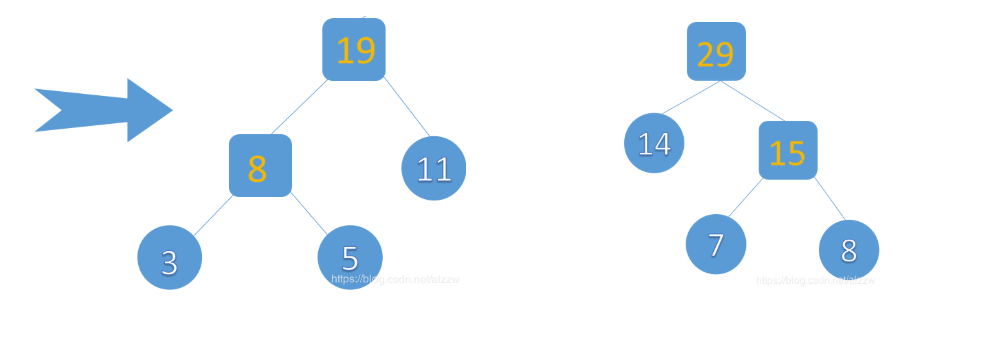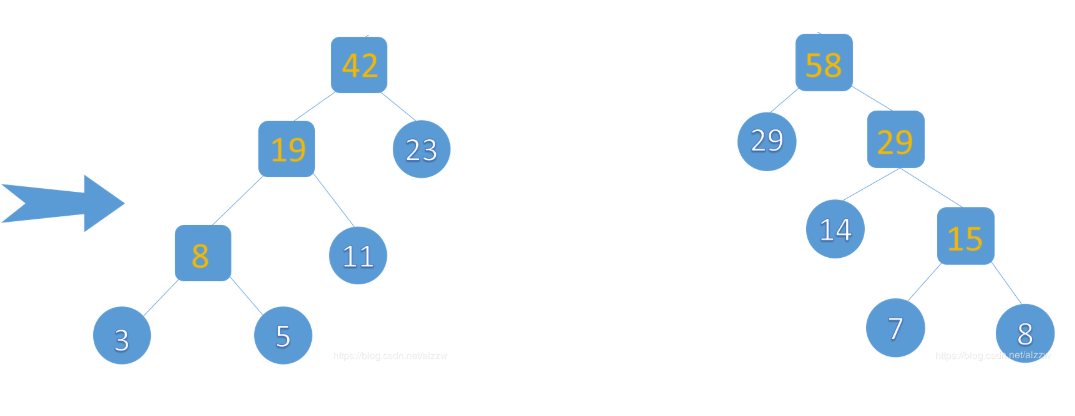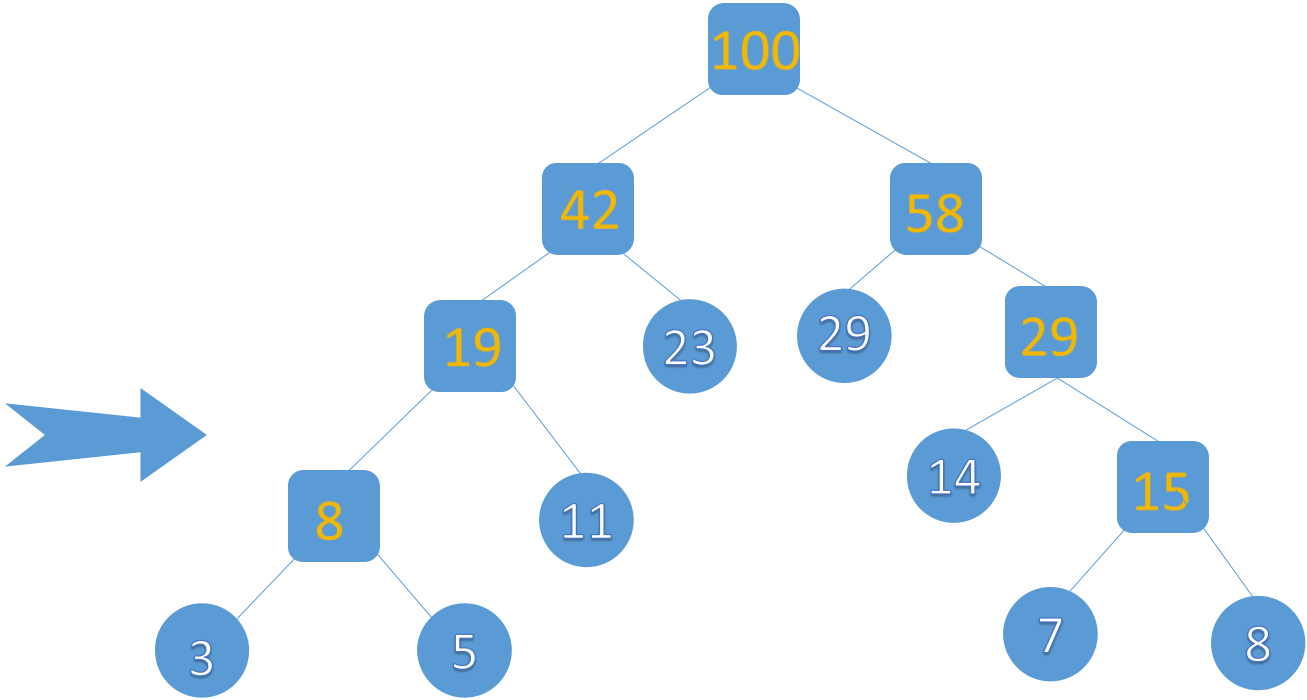特点：n1=0

所以：n = n0+n1+n2

= n0+n2

= 2n0-1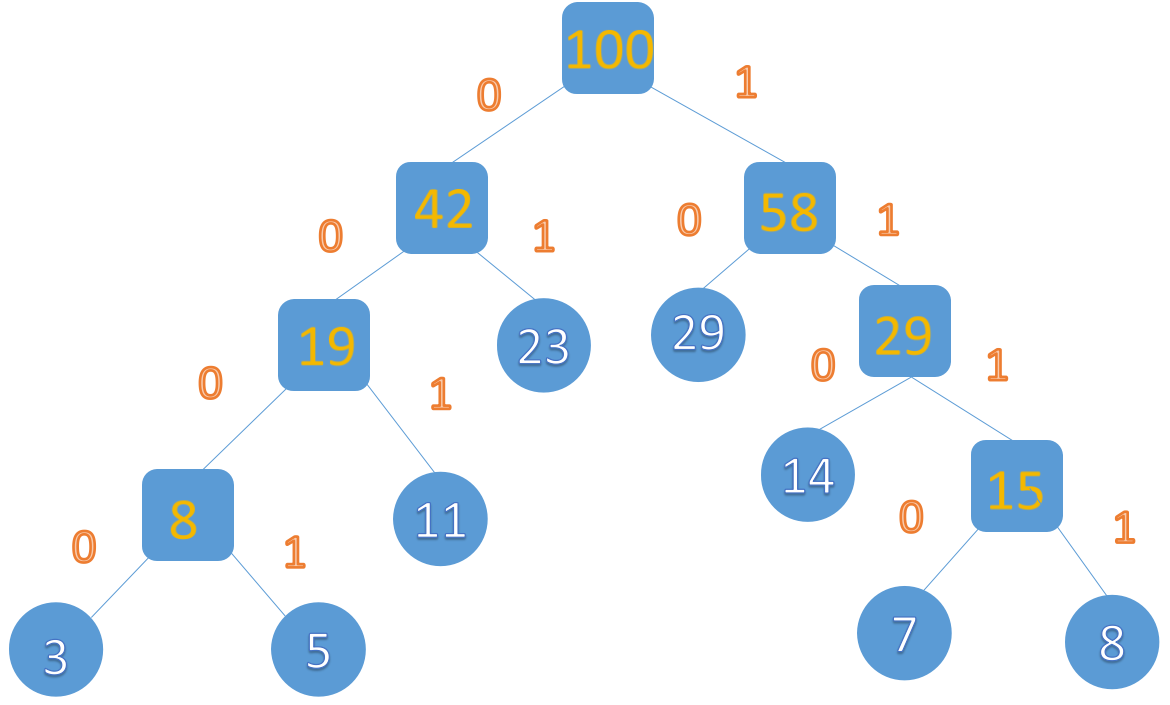3：（0000） 5：（0001） 11：（001） 23：（01） 8：（1111） 7：（1110） 14：（110） 29：（10）

例如，有4个字符的编码如下： 100，001，0，1

A．01,0000,0001,001,1

B．011,000,001,010,1

C．000,001,010,011,100

D．0,100,110,1110,1100

应用：

（1）发送方传输的二进制编码，到接收方解码后必须具有唯一性，即解码结果与发送方发送的电文完全一样；
（2）发送的二进制编码尽可能地短。下面我们介绍两种编码的方式。

1. 等长编码
这种编码方式的特点是每个字符的编码长度相同（编码长度就是每个编码所含的二进制位数）。假设字符集只含有4个字符A，B，C，D，用二进制两位表示的编码分别为00，01，10，11。若现在有一段电文为：ABACCDA，则应发送二进制序列：00010010101100，总长度为14位。当接收方接收到这段电文后，将按两位一段进行译码。这种编码的特点是译码简单且具有唯一性，但编码长度并不是最短的。

2. 不等长编码
在传送电文时，为了使其二进制位数尽可能地少，可以将每个字符的编码设计为不等长的，使用频度较高的字符分配一个相对比较短的编码，使用频度较低的字符分配一个比较长的编码。例如，可以为A，B，C，D四个字符分别分配0，00，1，01，并可将上述电文用二进制序列：000011010发送，其长度只有9个二进制位，但随之带来了一个问题，接收方接到这段电文后无法进行译码，因为无法断定前面4个0是4个A，1个B、2个A，还是2个B，即译码不唯一，因此这种编码方法不可使用。

3.压缩

代码：

#include <stdio.h>
#include <stdlib.h>
#include <string.h>
#include <malloc.h>
#include <iostream>
using namespace std;
#define infinity 10000
typedef struct
{
unsigned int weight;
unsigned int parent, lchild, rchild;
}HTNode, *HuffmanTree;
typedef char **HuffmanCode;
int Min(HuffmanTree t, int n);
void Select(HuffmanTree *t, int n, int *s1, int *s2);
void HuffmanCoding(HuffmanTree *HT, HuffmanCode *HC, int *w, int n);
void main()
{
HuffmanTree HT;
HuffmanCode HC;
int *w, n, i;
cout << "请输入叶子结点的个数：";
cin >> n;
w = (int *)malloc(n*sizeof(int));
for (i = 0; i < n;i++)
{
cout << "请输入第" << i + 1 << "个结点的权值:";
cin >> w[i];
}
HuffmanCoding(&HT, &HC, w, n);
for (i = 1; i <= n;i++)
{
cout << "权值为" << w[i - 1] << "的哈夫曼编码：";
cout << HC[i] << endl;
}
for (i = 1; i <= n;i++)
{
free(HC[i]);
}
free(HC);
free(HT);

system("pause");
}
void HuffmanCoding(HuffmanTree *HT, HuffmanCode *HC, int *w, int n)
{
int m, i, s1, s2, start;
unsigned int c, f;
HuffmanTree p;
char *cd;
if (n<=1)
{
return;

}
m = 2 * n - 1;
*HT = (HuffmanTree)malloc((m + 1)*sizeof(HTNode));
for (p = *HT + 1, i = 1; i <= n;i++,p++,w++)
{
(*p).weight = *w;
(*p).parent = 0;
(*p).rchild = 0;
(*p).lchild = 0;
}

for (; i <= m;i++,p++)
{
(*p).parent = 0;
}
for (i = n + 1; i <= m;++i)
{
Select(HT, i - 1, &s1, &s2);
(*HT)[s1].parent = (*HT)[s2].parent = i;
(*HT)[i].lchild = s1;
(*HT)[i].rchild = s2;
(*HT)[i].weight = (*HT)[s1].weight + (*HT)[s2].weight;

}
*HC = (HuffmanCode)malloc((n + 1)*sizeof(char*));
cd = (char*)malloc(n*sizeof(char));
cd[n - 1] = '\0';
for (i = 1; i <= n;i++)
{
start = n - 1;
for (c = i, f = (*HT)[i].parent; f != 0;c=f,f=(*HT)[f].parent)
{
if ((*HT)[f].lchild==c)
{
cd[--start] = '0';
}
else
{
cd[--start] = '1';
}
(*HC)[i] = (char*)malloc((n - start)*sizeof(char));
strcpy((*HC)[i], &cd[start]);
}

}
free(cd);
}

int Min(HuffmanTree t, int n)
{
int i, flag;
int f = infinity;
for (i = 1; i <= n;i++)
{
if (t[i].weight<f&&t[i].parent==0)
{
f = t[i].weight, flag = i;

}

}
t[flag].parent = 1;
return flag;
}

void Select(HuffmanTree *t, int n, int *s1, int *s2)
{
int x;
*s1 = Min(*t, n);
*s2 = Min(*t, n);
if ((*t)[*s1].weight>(*t)[*s2].weight)
{
x = *s1;
*s1 = *s2;
*s2 = x;
}
}
11-22266511-301808
01-29358
02-18507
03-221728
08-02628
06-013832
11-226568
09-21179
03-112004
04-07343
10-19653
04-24809
11-18266
05-083万+
03-30230
07-011212
11-294万+
03-1113万+¥2 ¥4 ¥6 ¥10 ¥20余额支付 (余额：-- )扫码支付获取中扫码支付点击重新获取扫码支付1.余额是钱包充值的虚拟货币，按照1:1的比例进行支付金额的抵扣。
2.余额无法直接购买下载，可以购买VIP、C币套餐、付费专栏及课程。余额充值